• 专题五 数据分析与多项式计算 一 数据统计分析 本部分主要包括求最大元素与最小元素、求平均值与中值、求和与求积、累加和与累乘积、求标准差与相关系数、排序等。 1. 求矩阵的最大元素和最小元素 max()：求...
专题五  数据分析与多项式计算

一    数据统计分析

本部分主要包括求最大元素与最小元素、求平均值与中值、求和与求积、累加和与累乘积、求标准差与相关系数、排序等。

1. 求矩阵的最大元素和最小元素

max()：求向量或矩阵的最大元素。
min()：求向量或矩阵的最小元素。
上述两函数的调用格式相同。以下以max函数为例进行介绍，min函数的用法与以下介绍相同。

当函数参数为向量时，函数有两种调用格式：

y=max(X)：返回向量X的最大值存入y。如果X中包含复数元素，则按模取最大值。
[y, k]=max(X)：返回向量X的最大值存入y，最大值元素的序号存入k。如果X中包含复数元素，则按模取最大值。
举个例子：求向量x的最大元素及最大元素的位置。

clear; clc;
x = [-43, 72, 9, 16, 23, 47];
[y, k] = max(x)

运行结果显示y=72, k=2。

当函数参数为矩阵时，函数有三种调用格式：

max(A)：返回一个行向量。向量的第i个元素是矩阵A的第i列上的最大值。
[Y, U]=max(A)：返回行向量Y和U。向量Y记录矩阵A的每列的最大值，向量U记录每列最大值元素的行号。
max(A, [], dim)：dim取1或者2。当dim取1时，该函数的功能和max(A)完全相同，按列取最大值；当dim取2时，按行取最大值，该函数返回一个列向量，其第i个元素是矩阵A的第i行上的最大值。
举个例子：求矩阵A的每行及每列的最大元素，并求整个矩阵的最大元素。

clear; clc;
A = [13, -56, 78; 25, 63, -235; 78, 25, 563; 1, 0, -1];

% 求矩阵A的每列的最大元素及最大元素的位置
[y1, k1] = max(A);

% 求矩阵A的每行的最大元素及最大元素的位置
[y2, k2] = max(A, [], 2);
% or  [y2, k2] = max(A');

% 求整个矩阵的最大元素
y = max(max(A));
% or   y = max(y1);   or   y = max(y2); 

工作空间中保存的变量如下：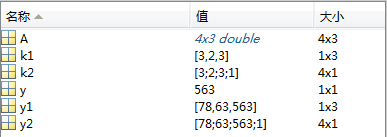用什么方法只调用一次max函数就能求得整个矩阵的最大值？

clear; clc;
A = [13, -56, 78; 25, 63, -235; 78, 25, 563; 1, 0, -1];
[y, k] = max(A(:)); % 采用A(:)的方法将矩阵元素按列堆叠，形成一个列向量

运行结果显示y=563, k=11。

2. 求矩阵的平均值和中值

平均值指的是算术平均值，即每项数据之和除以数据的项数。
中值：指在数据序列中，其值的大小处在中间的元素。如果数据个数为奇数，则取值为大小位于中间的元素；如果数据个数为偶数，则取中间两个元素的平均值。
有了平均值，为什么还要中值？

因为平均值容易受少数极端数据的影响。

在MATLAB中求矩阵的平均值和中值：

mean()：求算术平均值。
median()：求中值。
上述两个函数的调用方式与max函数相似。

3. 求和与求积

在MATLAB中，对向量和矩阵进行求和与求积的函数如下：

sum()：求和函数。
prod()：求积函数。
上述两个函数的调用方式与max函数相似。

4. 累加和与累乘积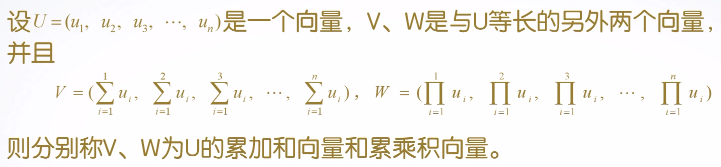在MATLAB中，对向量和矩阵进行累加和与累乘积的函数如下：

cumsum()：累加和函数。
cumprod()：累乘积函数。
举个例子：求向量X=[1,2,3,4,5,6,7,8,9,10]的积与累乘积。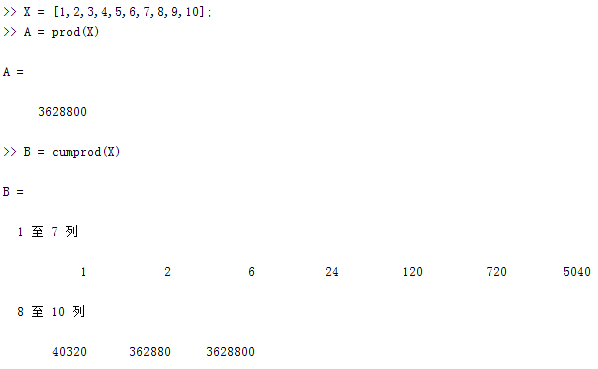5. 标准差与相关系数

标准差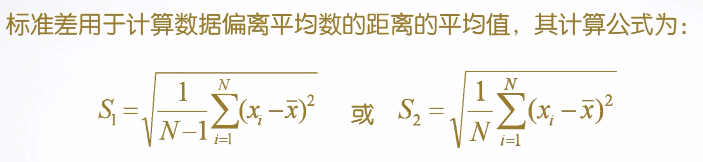其中，S1称为样本标准差，S2称为总体标准差。在MATLAB中用于计算数据序列标准差的函数是std()。std函数有3种调用格式：

std(X)：计算向量X的标准差。
std(A)：计算矩阵A的各列的标准差。
std(A,flag,dim)：其中flag取值为0或1。当flag=0时，按S1所列公式计算样本标准差；当flag=1时，按S2所列公式计算总体标准差。dim的用法与max函数中相同。默认情况下，flag=0, dim=1（即计算各列的样本标准差）。
相关系数r越接近于0，说明两组数据序列越不相关；|r|越接近于1，说明两组数据序列越相关。在MATLAB中计算两组数据序列之间相关系数的函数是corrcoef()。corrcoef函数有两种调用格式：

corrcoef(A)：返回由矩阵A所形成的的一个相关系数矩阵。其中，第i行第j列的元素表示原矩阵A中第i列与第j列之间的相关系数。
corrcoef(X,Y)：这里的X,Y是向量。它们与corrcoef([X,Y])的作用一样，用于求向量X,Y之间的相关系数。
6. 排序

在MATLAB中采用函数sort()对向量或矩阵进行排序。调用格式如下：

sort(X)：对向量X按升序排序。
[Y, I] = sort(A, dim, mode)：其中，dim指明对矩阵A的列还是行进行排序。mode指明按升序还是降序进行排序，若取'ascend'，则按升序排序，若取'descend'，则按降序排序，默认情况下，采用升序排序。输出参数中，Y是排序后的矩阵，而I记录了Y中的元素在A中的位置。
------------------------------------------------------------------------------------------------------------------------

二    多项式计算

1. 多项式的表示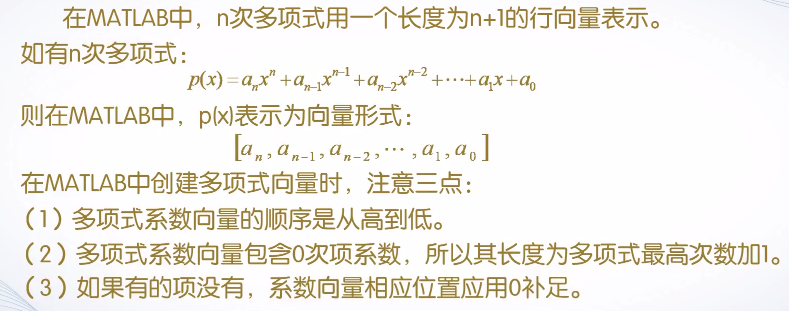2. 多项式的四则运算

加减运算直接用系数向量做加减。乘法用函数conv()，除法采用函数deconv()。用时具体查。

3. 多项式的求导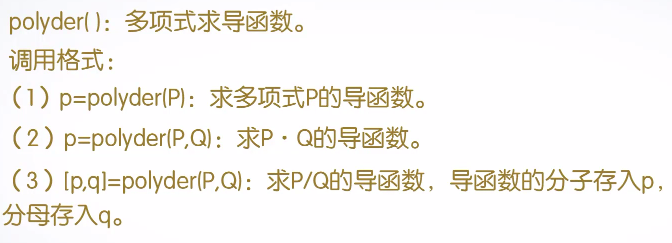4.多项式的求值

采用的函数如下：

polyval(p,x)：代数多项式求值。
polyvalm(p,x)：矩阵多项式求值。
polyval(p,x)中，p为多项式系数向量，x可以是标量，向量或矩阵。若x是标量，则求多项式在该点的值；若x为向量或矩阵，则对向量或矩阵中的每个元素求多项式的值。

polyvalm(p,x)要求x为方阵，以方阵为自变量求多项式的值。（这里需要理解以方阵为自变量的意思）

当x是方阵时，polyvalm(p,x)与polyval(p,x)的含义是不同的。polyval(p,x)是对方阵x中每个元素求多项式的值；而polyvalm(p,x)不同。例如：设x是方阵，p代表多项式x³-5x²+8，那么polyvalm(p,x)的含义是x*x*x-5*x*x+8*eye(size(x))；而polyval(p,x)的含义是x.*x.*x-5*x.*x+8*ones(size(x))。

5. 多项式求根

采用函数roots(p)，其中p是多项式的系数向量。若已知多项式的全部根，则可以用poly函数建立起该多项式，其调用格式为：p=poly(x)。

------------------------------------------------------------------------------------------------------------------------

三    数据插值

数据插值可以根据有限个点的取值状况，合理估算出附近其他点的取值，从而节约大量的实验和测试资源，节省大量的人力，物力和财力。

interp1()：一维插值函数。

其调用格式：Y1=interp1(X, Y, X1, method)，根据X, Y的值，计算函数在X1处的值。其中，X, Y是两个等长的已知向量，分别表示采样点和采样值。X1是一个向量或标量，表示要插值的点。输出参数Y1是一个与X1等长的插值结果。method表示某种特定的插值方法，method取值不同，所得到的结果也不同。method常用的取值有以下4种：

linear：线性插值，这是默认的方法。将与插值点靠近的两个数据点用直线连接，然后在直线上选取对应插值点的数据。
nearest：最近点插值。选择最近样本点的值作为插值数据。如果插值点与前后两点距离相等，则选择后一个样本点的值作为插值数据。
pchip：分段3次埃米尔特插值。采用分段3次多项式，除满足插值条件，还需满足在若干节点处相邻段插值函数的一阶导数相等，使得曲线光滑的同时，还具有保形性。
spline：3次样条插值。每个分段内构造一个3次多项式，使其插值函数除满足插值条件外，还要求在各节点处具有连续的一阶和二阶导数。
为什么这两种插值方法都用3次多项式而不使用更高次的多项式？

因为多项式次数并非越高越好。次数越高，越容易产生震荡而偏离原函数，这种现象称为龙格(Runge)现象。

interp2()：二维插值函数。

其调用格式：Z1=interp2(X, Y, Z, X1, Y1, method)，其中，X, Y是两个向量，表示两个参数的采样点，Z是采样点对应的函数值。X1, Y1是两个标量或者向量，表示要插值的点。二维插值函数中的method除了不能使用pchip插值以外，其余的与一维插值函数相同。

------------------------------------------------------------------------------------------------------------------------

四    曲线拟合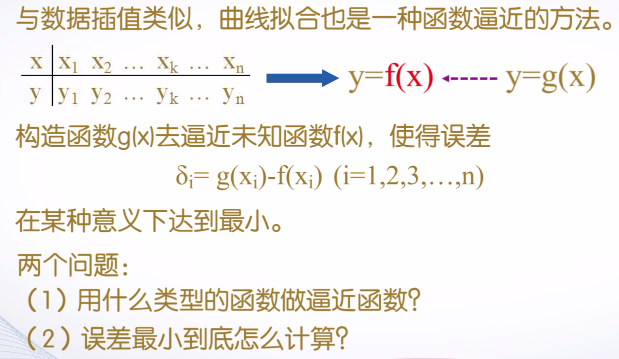一般是采用多项式函数做逼近函数，根据最小二乘法计算最小误差。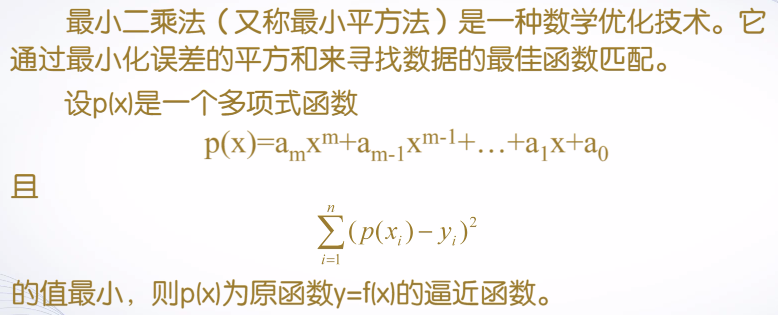在MATLAB中用于多项式拟合的函数是polyfit函数，该函数的功能是求得最小二乘拟合多项式的系数，polyfit函数有3种调用格式：

P=polyfit(X, Y, m)
[P, S]=polyfit(X, Y, m)
[P, S, mu]=polyfit(X, Y, m)
根据样本数据X和Y，产生一个m次多项式P及其在采样点误差数据S，mu是一个二元向量，mu(1)是mean(X)，mu(2)是std(X)。

曲线拟合的三个功能：估算数据、预测趋势、总结规律。

举例1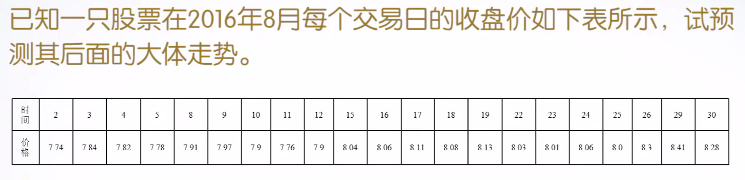首先，绘制该股票数据的散点图，可以看出：数据点跳动幅度大，不稳定，难以看出规律。

close; clear; clc;
x = [2, 3, 4, 5, 8, 9, 10, 11, 12, 15, 16, ...
17, 18, 19, 22, 23, 24, 25, 26, 29, 30];   % 交易日
y = [7.74, 7.84, 7.82, 7.78, 7.91, 7.97, 7.9, ...
7.76, 7.9, 8.04, 8.06, 8.11, 8.08, 8.13, ...
8.03, 8.01, 8.06, 8.0, 8.3, 8.41, 8.28];
figure('Name', '图1');
plot(x, y, '*');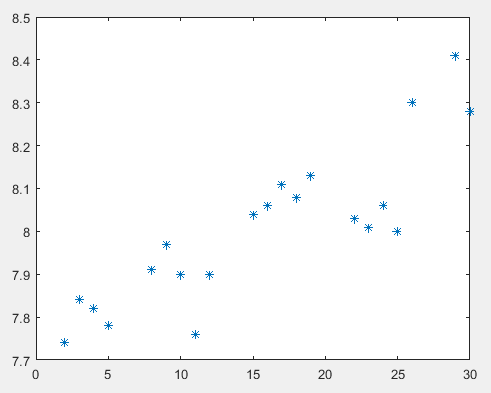继续输入命令，进行多项式拟合，选择常用的3次多项式，再绘制散点与拟合曲线图。

p = polyfit(x, y, 3);
plot(x, y, '*', x, polyval(p, x));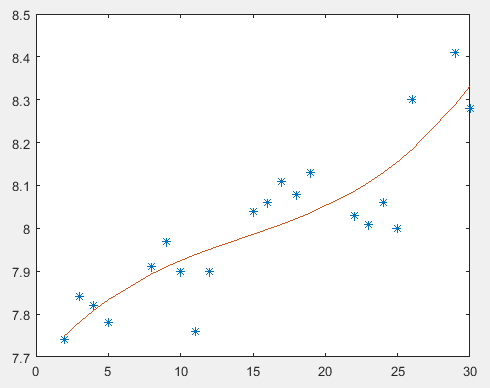预测未来3个交易日的股票数据。

x1 = [x, 31, 32, 33];
plot(x, y, '*', x1, polyval(p, x1)); 

数据插值与曲线拟合的比较

相同点：

都属于函数逼近方法。
都能根据有限的离散样本数据，去估算其他数据。
不同点：

实现方法不同。数据插值要求逼近函数经过样本点，曲线拟合不要求逼近函数经过样本点，只要求总体误差最小。
结果形式不同。数据插值没有统一的逼近函数，曲线拟合用一个函数进行整体逼近，有确定的函数表达式。
侧重点不同。数据插值一般是估算区间内的插值点数据，曲线拟合不仅可以估算区间内插值点的数据，还可以估算区间外的插值点数据。
应用场合不同。如果样本数据为精确数据，适合采用数据插值的方法；如果样本数据为统计数据或存在误差，则适合采用曲线拟合的方法。

展开全文• 这篇文章介绍二元域GF(2)上的不可约多项式计算方法，并用Matlab程序计算不可约多项式.
1 二元域 GF(2)$GF(2)$ 上的不可约多项式

二元域 GF(2)={0,1}$GF(2)=\{0,1\}$ 上的运算规则如下：

加法：+$+$
0
1

0
0
1

1
1
0

乘法：⋅$\cdot$
0
1

0
0
0

1
0
1

二元域 GF(2)$GF(2)$ 上的多项式具有形式
p(x)=anxn+an−1xn−1+⋯+a1x+a0p(x)=a_{n}x^{n}+a_{n-1}x^{n-1}+\cdots+a_{1}x+a_{0}
其中，ai∈GF(2), 0≤i≤n$a_{i}\in GF(2),\ 0\leq i\leq n$.

定义：一个次数大于等于 1$1$ 的多项式称为 不可约多的，如果它不能被分解为两个次数大于等于 1$1$ 的多项式的乘积.

显然，在二元域 GF(2)$GF(2)$ 上，一次多项式 p(x)=x+a0$p(x)=x+a_0$ 是不可约多项式；次数大于 1$1$ 并且常数项 a0=0$a_{0}=0$ 的多项式均为可约多项式（因为有一次因式 x$x$），从而次数大于 1$1$ 的多项式是不可约多项式的必要条件是常数项 a0=1$a_{0}=1$.

检查 GF(2)$GF(2)$ 上的多项式是否可约：设 p(x)=anxn+an−1xn−1+⋯+a1x+a0$p(x)=a_{n}x^{n}+a_{n-1}x^{n-1}+\cdots+a_{1}x+a_{0}$ 为 n$n$ 次多项式.
(a) 若 n=1$n=1$，则 p(x)$p(x)$ 为不可约多项式.
(b) 若 n>1$n>1$，
(b.1) 若 a0=0$a_{0}=0$，则 p(x)$p(x)$ 为可约多项式.
(b.2) 若 a1=1$a_{1}=1$，则用所有次数 ⌊n2⌋$\lfloor\frac{n}{2}\rfloor$ 的（不可约）多项式 g(x)$g(x)$ 除 p(x)$p(x)$. 若存在一个 g(x)$g(x)$ 使得 g(x)|p(x)$g(x)|p(x)$，则 p(x)$p(x)$ 为可约多项式，否则为不可约多项式.

2 二元域 GF(2)$GF(2)$ 上多项式的表示

多项式在计算机中常用其系数数组来表示. 自然地，二元域 GF(2)$GF(2)$ 上的一个 n$n$ 多项式
p(x)=anxn+an−1xn−1+⋯+a1x+a0p(x)=a_{n}x^{n}+a_{n-1}x^{n-1}+\cdots+a_{1}x+a_{0}
可以表示为一个 GF(2)$GF(2)$ 上的 n+1$n+1$ 维向量
P=(an,an−1,…,a1,a0)∈GFn+1(2)P=(a_{n},a_{n-1},\dots,a_{1},a_{0})\in GF^{n+1}(2)
因此，多项式环 GF(2)[x]$GF(2)[x]$ 和 GF(2)$GF(2)$ 上的无穷维向量空间 GF∞(2)$GF^{\infty}(2)$ 之间存在“1-1”对应关系.

更进一步，任意一个 GF(2)$GF(2)$ 上的向量可以转化为一个非负整数：
(an,an−1,…,a1,a0)→∑i=0nai2i(a_{n},a_{n-1},\dots,a_{1},a_{0})\rightarrow \sum^{n}_{i=0}a_{i}2^{i}
显然，这也是一个“1-1”对应关系. 从而，二元域 GF(2)$GF(2)$ 上的一个多项式 p(x)=anxn+an−1xn−1+⋯+a1x+a0$p(x)=a_{n}x^{n}+a_{n-1}x^{n-1}+\cdots+a_{1}x+a_{0}$ 与一个非负整数 ∑ni=0ai2i$\sum^{n}_{i=0}a_{i}2^{i}$ 相对应，我们得到“1-1”对应关系：
GF(2)[x]→Z∗GF(2)[x]\rightarrow\mathbb{Z}^{*}
p(x)=∑i=0naixi↦p(2)=∑i=0nai2ip(x)=\sum^{n}_{i=0}a_{i}x^{i}\mapsto p(2)=\sum^{n}_{i=0}a_{i}2^{i}

3 判断 GF(2)$GF(2)$ 上两个多项式的整除关系

在下面的 Matlab 函数判断 GF(2)$GF(2)$ 上的一个多项式是否能被另一个多项式整除，其中，多项式表示为由 ‘0’ 和 ‘1’ 组成的字符串的形式. 例如，多项式 f(D)=D3+D+1$f(D)=D^{3}+D+1$ 表示为 ′1011′$'1011'$.

function b = isDivisible(f,g)
% 判断二元域 GF(2) 上两个多项式的整除关系
% 若 'f' 可以被 'g' 整除，则返回 1；否则返回 0;
% 输入
% f: 被除式，由 '0' 和 '1' 组成的字符串来表示
% g: 除式，由 '0' 和 '1' 组成的字符串来表示
% 输出
% 若 'g' 整除 'f'，则输出 1；否则，输出 0
%
% 如果被除式为 0，则返回 true (1)
if isempty(find(f,'1'))
b = true;
return;
end
% 去除高次的 0 系数
pos = find(f=='1',1);
f = f(pos:length(f));
len_f = length(f);
% 检查除式是否为零
if isempty(find(g=='1'))
error('Error: f is divided by 0')
end
% 去除高次的 0 系数
pos = find(g=='1',1);
g = g(pos:length(g));
len_g = length(g);
% 若被除式的次数小于除式的次数，返回不可整除
b = false;
if len_f < len_g
return;
end
% 除法
for i = 1:len_f-len_g+1
if f(i) == '0'
continue;
end
for j=1:len_g
if f(i+j-1) == g(j)
f(i+j-1) = '0';
else
f(i+j-1) = '1';
end
end
end
% 检查余式是否为 0
b = true;
for i=len_f-len_g+1:len_f
if f(i) == '1'
b = false;
break;
end
end

end


例：判断在 GF(2)$GF(2)$ 上多项式 x3+x2+x+1$x^{3}+x^{2}+x+1$ 和 x3+1$x^{3}+1$ 能否被多项式 x2+1$x^{2}+1$ 整除.

>> isDivisible('1111','101')

ans =

1

>> isDivisible('1001','101')

ans =

0


注：在Matlab中，函数 dec2bin 和 bin2dec 分别将一个整数转化为’01’字符数组和将一个’01’字符数组转化为整数. 利用前一个函数，我们可以用整数来表示 GF(2)$GF(2)$ 上的多项式，并把上面函数的输入类型改为两个整数；后一个函数将在下一节使用.

4 计算 GF(2)$GF(2)$ 上的不可约多项式

下面的 Matlab 函数计算所有 GF(2)$GF(2)$ 上次数不超过 n$n$ 次的不可约多项式.

function [IrrPolys,Nums] = AllIrrPolys(n)
% 计算二元域 GF(2) 上所有次数不超过 'n' 的不可约多项式
% 输入
% n: 多项式的次数
% 输出
% IrrPolys: 所有次数不超过 'n' 的不可约多项式
% Nums: 各次的不可约多项式的个数
%
Nums = zeros(1,n); % '1' 至 'n' 次不可约多项式的个数
% '1' 次不可约多项式
IrrPolys = [bin2dec('10'),bin2dec('11')];
Nums(1) = 2;
total_num = 2;
% '2' 至 'n' 次不可约多项式
for d = 2:n
cnt = 0;
for k = (2^d+1):2:(2^(d+1)-1)
isDiv = false;
for s = 1:floor(d/2)
off_set = sum(Nums(1:s-1));
for t = 1:Nums(s)
isDiv = isDivisible(dec2bin(k),dec2bin(IrrPolys(off_set+t)));
if isDiv
break;
end
end
if isDiv
break;
end
end
if ~isDiv
total_num = total_num + 1;
IrrPolys(total_num) = k;
cnt = cnt + 1;
end
end
Nums(d) = cnt;
end

end


注1：在函数中，GF(2)$GF(2)$ 上的多项式用其对应的正整数来表示.

注2：所有不可约多项式都保存在数组 IrrPolys 中. 可用命令 IrrPolys(sum(Nums(1:k-1))+1:sum(Nums(1:k))) 列出所有次数为 k 的不可约多项式.

注3： GF(2)$GF(2)$ 的 n$n$ 次不可约多项式的个数为
N(n)=1n∑d|nμ(d)2n/dN(n)=\frac{1}{n}\sum_{d|n}\mu(d)2^{n/d}
其中 μ$\mu$ 为Moebius函数，定义为
μ(m)=⎧⎩⎨1(−1)k0如果m=1如果m=p1p2⋯pk,其中p1,p2,…,pk为互不相同的素数其它\mu(m)=\begin{cases}1 & 如果 m=1\\ (-1)^{k} & 如果 m=p_{1}p_{2}\cdots p_{k},其中 p_{1},p_{2},\dots,p_{k}为互不相同的素数\\ 0 & 其它\end{cases}

例：计算所有 GF(2)$GF(2)$ 上次数不超过 5$5$ 次的不可约多项式，并列出所有 4$4$ 次不可约多项式.

>> [IrrPolys,Nums] = AllIrrPolys(5)

IrrPolys =

Columns 1 through 13

2 3 7 11 13 19 25 31 37 41 47 55 59

Column 14

61

Nums =

2 1 2 3 6

>> IrrPolys(sum(Nums(1:4-1))+1:sum(Nums(1:4)))

ans =

19 25 31

展开全文程序
• 本期我打算给大家介绍一下使用matlab多项式进行的一些基本运算，如求根、求导、四则运算等或者还有其他一些简单的数据分析的内容。 正文开始 多项式及其建立 在运算中我们经常接触到的就是所谓的多项式，比如很...
前期回顾

上一期中，我们学了下面的知识：

定积分的基本概念和一些简单的几何意义。
使用 int 函数计算不定积分。
使用 int 函数计算定积分。
本期内容

本期我打算给大家介绍一下使用matlab对多项式进行的一些基本运算，如求根、求导、四则运算等或者还有其他一些简单的数据分析的内容。

正文开始

多项式及其建立

在运算中我们经常接触到的就是所谓的多项式，比如很常见的一个多项式：

这里我们就说这是一个x的多项式，最高次是2次，常数项是3，二次项的系数是1，一次项的系数是2，相信这些大家都知道，就不再赘述了。

那么在matlab中，如何建立一个多项式呢？

通过系数建立多项式

如果在演草纸上，我们需要进行如下的步骤：

确定最高次项是几次。
确定各个次项的系数。
常数项是多少。
确定了上面的东西，就基本上可以确定一个多项式了，为了方便，我们使用程序表示这样一个多项式： ，那么上述步骤在程序中是这样表示出来的：

clc;clear;
%% 使用matlab建立多项式
p = [4 3 2 1 1]; %确定各项系数，和常数项。放在一个数组里面。
y = poly2sym(p); %创建目标多项式，这个函数返回了一个符号变量，即我们的函数
disp(y); %显示y.

PS: 如果需要定义一个特殊的函数比如  ，那么我们可以认为3次、2次、1次系数都为0，常数项也为0，写入数组即可。

上面的程序运行结果如图所示：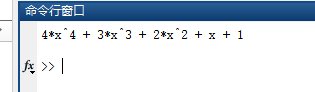通过已知方程的根建立多项式

假设现在已有方程根： ，要求得到这个方程的式子，对于一元二次方程来讲，一个方法是：伟达定理，利用根与系数的关系，在matlab中，这种问题也可以得到解决，只需要一个函数即可：

clc;clear;
%% 使用根建立多项式
r = [1 2 3]; %随意编一些根
p = poly(r); %调用求多项式的函数
y = poly2sym(p); %得到多项式的符号变量
disp(y);

运行结果如图（我还不知道有没有可能求不出来这个对应方程，没试过哈哈哈哈，我猜应该是只要给出一套根，总能给出一个对应方程吧）：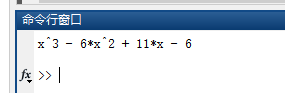对多项式进行求导

求导即求多项式的导数，一般来讲，习惯上我们把 y = y(x) 对x求导函数直接简称为求导了，但是，我们求导时，一定要明确谁在对谁求导！！！在下文中，不做特殊说明的话，我们默认因变量是y，自变量是x，求导默认为 y对x求导，即求 。

导数的意义：

PS: 此部分是关于导数的一些基础知识，有相应基础的可以跳过，CSDN右边有目录结构，可以直接根据标题跳转。

导数，在某种程度上，我们可以理解为变化率，即 y 随着 x 的变化而变化的幅度、大小、快慢等，举个例子，我说我的速度是匀速，60km/h（60千米每小时），此时：自变量(x)是时间，因变量(y)是速度，我们可以很清楚的知道，我的速度是匀速（60km/h），所以我的速度(y)不随着时间(x)的变化而变化，所以我的速度变化率是0，所以此时 。在图形上面表示如图所示：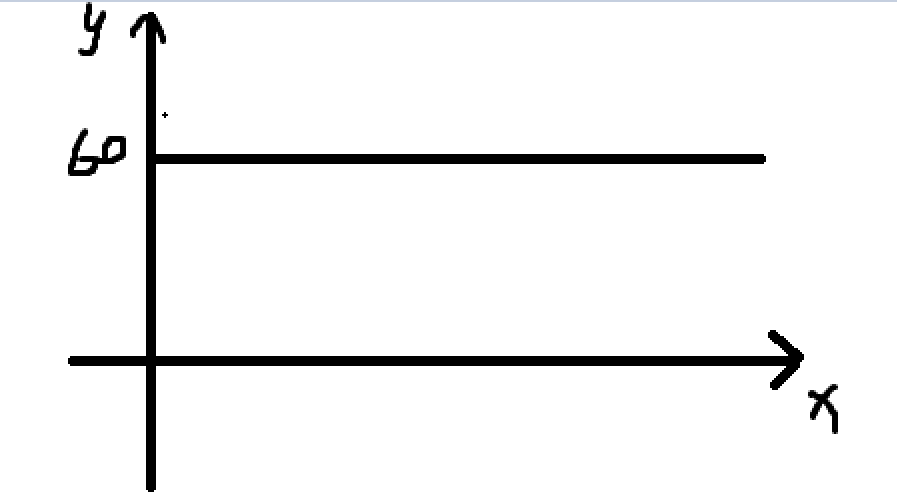下面假设我的速度不再是匀速，而是慢慢变快的，但是加快的速度是恒定的，都是每分钟加10km/h，比如刚起步是0，一分钟后是10km/h，两分钟后是20km/h.。。。。。类推，此时我们知道，我的速度(因变量y)随着时间(自变量x)是在慢慢改变的（并且还是均匀改变的），所以此时有一个变化率，即 10km/h/min，就是每分钟改变 10km/h的速度，（大家不用理会单位，这个不重要，重要的是知识点），在图形中是这样表示的：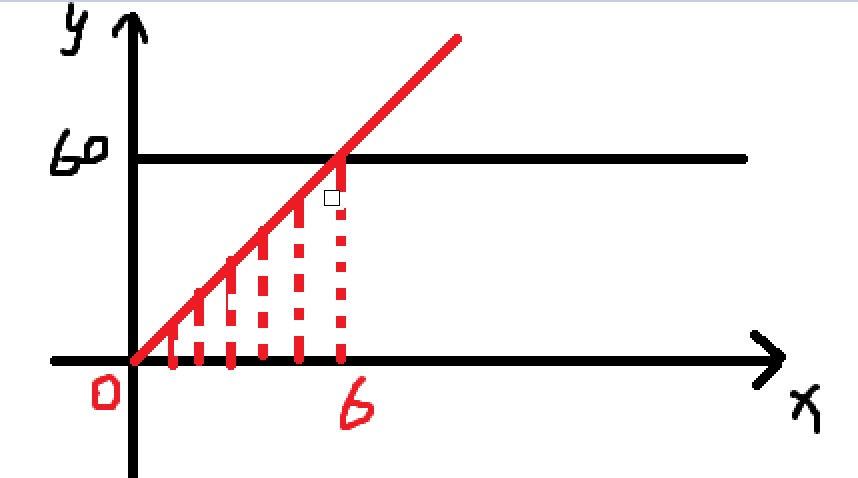在红色的图形中，就可以看到上面的变化的速度的例子。那么求导数就可以理解为是反映这个变化率的一个方式。

一些基本知识点(u和v都是x的函数，即 u = u(x), v = v(x)。)：

常数导数为0；（即变化率为0，不改变）
多项式求导公式： 。

理解上述知识点应该就可以理解今天的内容了，如果有什么不懂的，欢迎大家留言或者私信！。

使用matlab进行求导

代码如下：

clc; clear;
%% 对多项式进行求导
p = [4 3 2 1 1]; %建立我们的多项式
y = poly2sym(p); %得到多项式的符号变量
temp_str = sprintf('得到多项式:\ny = %s', y); %将式子格式化到一个字符串中
disp(temp_str); %显示
p_1 = polyder(p); %求一次导,注意polyder求导传递的是p而不是y
y_1 = poly2sym(p_1); %转化为符号变量
temp_str = sprintf('求导结果是:\ny_1 = %s\n求导后系数是:[%d %d %d %d]', y_1, p_1);
disp(temp_str);

运行结果如图所示：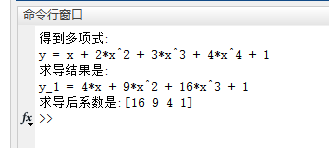y_1中的常数1来自于y中的x求导，y中的1求导后成为了0，就没了。

那么，这仅仅是一个多项式求导，乘积求导、商求导，请继续往下看：

乘积求导：

%% 乘积求导
clc; clear;
p_1 = [4 4 2];
p_2 = [2 4 5]; % 随意胡诌几个数
y_1 = poly2sym(p_1);
y_2 = poly2sym(p_2); % 转化为符号变量
temp_str = sprintf('原来的两个函数为：\n 1. y_1 = %s\n 2. y_2 = %s', y_1, y_2);
disp(temp_str);
res_p = polyder(p_1, p_2); % 乘积求导后的系数
res_y = poly2sym(res_p); %转化为符号变量
temp_str = sprintf('结果为：y = %s', res_y);
disp(temp_str);

运行结果如图所示：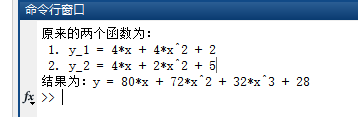商求导：

这个部分比较麻烦，商求导后返回值是有两个，因为很有可能出现求导后还是分数的形式，所以返回值是一个分子、一个分母，代码如下：

%% 商求导
clc; clear;
p_1 = [4 4 2];
p_2 = [2 4 5]; % 随意胡诌几个数
y_1 = poly2sym(p_1);
y_2 = poly2sym(p_2); % 转化为符号变量
temp_str = sprintf('原来的两个函数为：\n 1. y_1 = %s\n 2. y_2 = %s', y_1, y_2);
disp(temp_str);
[res_p_Num, res_p_Den] = polyder(p_1, p_2);
% 注意此处，与乘积求导几乎一样，只是返回值不同，
% 前面的是分子，后面的是分母
res_y_Num = poly2sym(res_p_Num);
res_y_Den = poly2sym(res_p_Den); %转化为符号变量
temp_str = sprintf('结果为：y = (%s)/(%s)', res_y_Num, res_y_Den);
disp(temp_str);

在商求导这个地方，所需要修改的仅仅是返回值而已，其他的地方几乎与乘积求导一致，还是很方便的。

运行结果如图所示：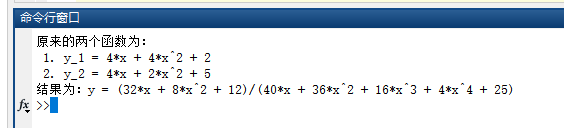结束语

今天我们学习了如下内容：

在matlab中使用已知系数、已知根简历方程多项式的方法。
求导的浅薄的基本概念。
在matlab中对多项式求导的一些函数，包括一些典型的求导类型：乘积求导、商求导等。
那么今天就介绍到这里！谢谢大家观看！如果有疑问，欢迎大家评论留言！

展开全文matlab matlab求导
• 多项式是比较容易计算和直观的一种算式,也是比较容易被感知的,这里介绍多项式的解法。 例如: p(x) = x^3+4*x^2-7*x-10 此多项式matlab的表示方法为: 多项式 p=[1 4 -7 -10]，其中的数值是多项式的各阶项（从...
多项式是比较容易计算和直观的一种算式,也是比较容易被感知的,这里介绍多项式的解法。例如:        p(x) = x^3+4*x^2-7*x-10此多项式的matlab的表示方法为:    多项式 p=[1 4 -7 -10]，其中的数值是多项式的各阶项（从高到低）的各个系数。如果要计算一个多项式可以使用指令完成,polyval直接做运算:[code]x=linspace(-1,3);p=[1 4 7 -10];v=polyval(p,x);[/code]多项式的加法、减法、乘法、除法例如有两个多项式:   a(x) = x^3+2x^2+3x+4   b(x) = x^3+4x^2+9x+16很显然的是加法、减法相对的比较的简单，但是乘法、除法就显得有些麻烦。需要借助与指令来完成，主要是乘法conv、除法deconv。二多项式相乘，在数学上等于二个阵列做旋积(convolution)运算。而二多项式相除就相 当于反旋积(de-convolution) 运算。deconv 函数，其语法稍有不同[q,r]=deconv(a,b)，其中q,r分别代表整除多项式及余数多项式。[code]%加法:a=[1 2 3 4]; b=[1 4 9 16];c = a+bc =     2     6    12    20[/code][code]%减法d = a-bd =     0    -2    -6   -12[/code][code]%乘法e=conv(a,b)e =1 6 20 50 75 84 64 [/code][code]%除法>> [f,r]=deconv(e,b)f =1 2 3 4r =0 0 0 0 0 0 0 % 因为是整除所以余数多项式的各系数皆为零 [/code]多项式的根视阶数而定，它的根可以有一个到数个，可能为实数也可能是复数。但在matlab下面求多项式的根就比较的简单，尤其是高介的多项式，我们不必要借助数值方法，而一概使用roots(p);只要输入多项式的各阶系数（以p代表）即可求解到对应的根。比如:x^2+3x+2=0我们知道它的两个根为-2，-1。那么使用matlab来确认一下就是:[code]>> p=[1 3 2];r=roots(p) r =    -2    -1[/code][code]%有实根与复根的多项式的解>> p=[1 -12 0 25 116]; % 注意二阶项系数为零须要输入，否则多项式的阶数就不对r=roots(p) % 有实数根及复数根 r =  11.7473             2.7028            -1.2251 + 1.4672i  -1.2251 - 1.4672i[/code]与 roots 相关的函数尚有 poly, real，这二个函数的用途是要验算求解的根展开能求得原多项式。[code]>> r=[-2 -1];pp=poly(r) % pp=(x+2)(x+1)=x^2+3x+2 pp =     1     3     2[/code]产生虚假根部的情况[code]>> pp=[1 7 12 9]; % 多项式>> r=roots(pp)r =-4.9395-1.0303 + 0.8721i-1.0303 - 0.8721i>> pp=poly(r) % 注意因计算的误差会有假虚部产生pp =1.0000 7.0000 12.0000 9.0000 + 0.0000i>> pp=real(pp) % 可以real将假虚部去除，将原多项式还原pp =1.0000 7.0000 12.0000 9.0000[/code]
展开全文C C++ C# F#
• MATLAB具有强大的数值计算功能。本章主要介绍几种常用的计算方法，包括方程求解、多项式计算、插值、基本统计和曲线拟合等。
• 第二章、插值理论（续）上一讲我们介绍了拉格朗日插值法和牛顿插值法，这两者都是对给定 个节点上的函数值来求出 次插值多项式，而现在我们的函数表上又新添加了 个节点上的导数，我们想让插值多项式的性质更加好，...
• 基于matlab7.0的matlab 第二章 matlab 数值计算PPT 介绍Matlab语言的数值运算功能，通过学习应该掌握： 如何创建矩阵 矩阵及数组运算 多项式运算 线性方程组 拟合与插值
• 主要介绍了三种差值方法：最近邻插值(nearest neighbor interpolation)：最简单，误差最大双线性插值(bilinear interpolation)：有低通滤波性质三次插值：多项式、B样条等，精度高但计算量大具体任务描述：读图...
• 主要介绍Lagrange插值和Newton插值，给出了Lagrange插值的matlab代码数值分析
• 本文主要翻译自这篇文章译者注★ 本文承接...”★ 接着介绍了群的阶数的概念，介绍计算椭圆曲线上的群的阶数的算法，并讨论群和子群的阶数的联系 ”★ 随后展示了如何用椭圆曲线上的任意一点，通过计算点的倍数的...
• 《数值计算方法与MATLAB应用》第l篇介绍了数值计算的基本方法和基本概念，包括算法与误差、非线性方程的求解以及线性方程组的直接解法和迭代解法、插值多项式的基本概念、曲线拟合方法、定积分的数值计算以及常微分...
• 多项式和有理分式运算 数据统计分析 数值积分 优化处理 高级又简单的程序环境： 命令行的交互式工作方式 程序文件方式工作，是一种面向对象的程序设计语言 扩充能力强，可以自定义工具箱 开放的源程序，可进行二...数据分析 数学建模
• 科学计算MATLAB语言 幻灯片 教学内容 第1讲介绍MATLAB概述与运算基础 第2讲介绍MATLAB程序设计 第3讲MATLAB文件操作 第4讲绘图形功能 第5讲线形代数中的数值计算问题 第6讲数据处理方法与多项式 第7讲...
• 在下面的介绍中主要从这几个方面介绍matlab基本的数值计算指令： 1.矩阵的创建 2.矩阵的运算 3.多项式的运算 4.线性插值1.矩阵的创建matlab中矩阵的创建主要有两种方式： 1.通过直接输入矩阵数据创建 2.通过...
• MATLAB在日常计算中的应用》由大量的MATLAB计算实例组成。通俗易懂，图文并茂，资料丰富，实用性强。提供大量MATLAB计算实例以及全部实例的M文件，方便读者使用。既适合初学者，也适合有一定MATLAB基础的爱好者或...
• ## 秦九韶算法介绍及MATLAB实现

万次阅读 多人点赞 2019-05-22 00:04:20
数值计算中算法设计好坏不但影响计算结果的精度，还可大量节省计算时间，减少运算次数和舍入误差，这也是算法设计中一个重要原则，下面以多项式求值为例，介绍数值计算中第一个常用的技巧——秦九韶算法或Horn...秦九韶算法 C语言 Java
• 第24章数值计算MATLAB实现 24.1矩阵代数的应用 24.2数学建模的应用 24.3优化设计的应用 24.4拟合分析的应用 24.5非线性方程的应用 24.6数值模型的应用 24.7美丽的分形图 24.8共线平动点 第25章提升小波...
• 多项式插值（一） 参考《数值分析与科学计算》一书。 matlab里有大量关于插值的命令。 1、介绍vander()和fliplr()两个与范德蒙有关的函数 >> x =[0 pi/2 pi 3*pi/2]；v =vander(x) v = ...
• 第6章 MATLAB数据分析与多项式计算 第7章 MATLAB解方程与函数极值 第8章 MATLAB数值积分与微分 第9章 MATLAB符号计算 第10章 MATLAB图形句柄 第11章 MATLAB图形用户界面设计 第12章 Simulink动态仿真集成环境 第13章...
• 一、本期介绍函数的求解有两种（1）已知x求y（2）已知y求x1.1已知x求y回想我们上一期，讲了多项式求解的方法，也是已知x求y。想一下，多项式不也是函数的一种吗，所以本期求解的方法同样适用于多项式，只不过比...
• 介绍MATLAB的基本知识-MATLAB程序设计,文件操作,绘图功能,线性代数中的数值计算,数据处理方法与多项式,符号计算......
• 前言前面介绍了Lagrange、Newton、Hermite插值，都能在一定问题上取得不错的结果，但是我们根据之前的插值余项公式计算，发现误差并不理想，我们还想进一步提升精度。直观上觉得，提升精度就多增加一些取值点，用更...
• 第1讲介绍MATLAB概述与运算基础 第2讲介绍MATLAB程序设计 第3讲MATLAB文件操作 第4讲绘图形功能 第5讲线形代数中的数值计算问题 第6讲数据处理方法与多项式 第7讲MATLAB符号计算 第8讲MATLAB的图形用户界面设计
• 一、Reinforcement Learning Toolbox介绍 强化学习工具箱使用强化学习算法（包括DQN，A2C和DDPG）为训练策略（policy）提供函数和模块。您可以使用这些策略为复杂的系统（例如，机器人和自治系统）搭建控制器和开发...强化学习 人工智能
• https://blog.csdn.net/JISANSAN/article/details/105370933中介绍了通过期望多项式计算状态反馈K的函数T2place，其实matlab自带状态反馈设计的函数place，不同的是直接用期望的极点进行计算，在不同的情形下可能...place函数
• 提出了基于Matlab的插值响应面法和BP神经网络响应面法, 介绍了其在Matlab环境下的实现方法, 并进行了三种方法的对比分析。Matlab语言基本元素是矩阵, 提供了各种矩阵的运算和操作, 其中包含结构可靠度计算中常用的...
• 以下介绍一点matlab编程的技巧。 嵌套计算 程序执行的速度取决于调用的子程序的个数和算法实现。通常希望子程序越少越好。嵌套可以有效地减少子程序。 一个典型的例子就是多项式的求值计算：p(x)=a3x3+a2x2+a1x+...
• 第2篇介绍机械工程领域的机构运动分析和设计、机械零部件设计、机械可靠性设计、机械优化分析和计算、机械制造工艺参数优化、实验数据的曲线拟合和多项式拟合等方面的多个实例，并通过建立数学模型，利用功能强大的...
• 主要介绍MATLAB的编程基础，包括MATLAB的变量、MATLAB的运算符、矩阵的创建及运算等；MATLAB的数值与符号功能，包括多项式分析、符号对象创建与分析；高级语言的科学计算功能，包括插值、拟合及方程的求解等；...# matlab多项式计算介绍matlab 订阅